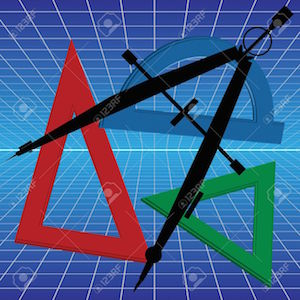# COURSE DESCRIPTIONAlgebra II expands the learning of foundational topics covered in Algebra, giving students a more complex understanding of the subject matter. The curriculum includes working with polynomial expressions and solving equations, including rational expressions. Concepts such as radicals, complex numbers, logarithms, and sequence and series are explained. The course concludes with the fundamentals of trigonometry including the unit circle, trigonometric graphs and translations, solving trigonometric equations and proving trigonometric identities as well as applications of trigonometry. Lessons are designed using a best practice model that includes scaffolding, vocabulary, prior knowledge, guided practice, independent practice, and written response opportunities for students to solve, analyze, and evaluate concepts. The lessons are designed according to the New York State Learning Standards.

# CHAPTERS

Ch.1 – Foundations of Trigonometry
• The Six Trig Ratios
• Identifying Angles in the Standard Position
• An Introduction to Periodic Functions
• The Co-Function and Reciprocal of Trig Functions
• Find the Value of Reciprocal Trig Functions

Ch.2 – The Unit Circle
• Circular Motion
• The Unit Circle Part I
• The Unit Circle Part II

Ch.3 – Trigonometric Functions
• Extending Trigonometry to All Angles
• The Tangent Function
• Secant, Cosecant, and Cotangent

• Find Value of Trig Expressions: Radians

Ch.5 – Graphs of Trigonometric Functions
• Analyzing the Graphs of Trigonometric Functions
• Graphs of Reciprocal Trig Functions

Ch.6 – Understanding Trigonometric Functions and Putting Them to Use
• Basic Trig Identities From Graphs
• Transformations of Trigonometric Functions
• Using Trig Functions to Model Physical Behavior

Ch.7 – Trigonometric Identities
• The Pythagorean Identity
• Solve Trigonometric Equations
• Prove Trigonometric Identities
• Sum & Difference Identities